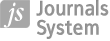Analyzing Mathematics Textbooks through a Constructive-Empirical Perspective on Abstraction: The Case of Pythagoras’ Theorem

More details
Hide details
1
Department of Mathematics, National Taiwan Normal University

Publication date: 2016-02-29

Corresponding author
Kai-Lin Yang

Department of Mathematics, National Taiwan Normal University, No. 88, Ting-Chou Rd Sec. 4, Department of Mathematics, 116 Taipei, Taiwan

EURASIA J. Math., Sci Tech. Ed 2016;12(4):913–930

KEYWORDS
TOPICS
ABSTRACT
Background:
This study aims at analyzing how Pythagoras’ theorem is handled in three versions of Taiwanese textbooks using a conceptual framework of a constructive-empirical perspective on abstraction, which comprises three key attributes: the generality of the object, the connectivity of the subject and the functionality of diagrams as the focused semiotic tool.

Material and methods:
This study used a content analysis methodology to analyze the selected topic, Pythagoras’ theorem. First, we classified texts by categories of text goals. The categories of text goals serve to identify analysis units. Second, each analysis unit was coded regarding each attribute. The last step was to enter the data into an Excel file for statistical analysis.

Results:
The results show that Taiwanese textbooks intended to develop the object through the variation among the levels of generality as well as between more and less familiar connections. The procedural diagrams were less provided than the conceptual diagrams, and the diagrams required more processing were less provided than the diagrams required less processing. Nonetheless, there were differences among the three textbooks.

Conclusions:
On the basis of similarities and differences, we discuss issues related to the learning and teaching of mathematics.

 eISSN: 1305-8223 ISSN: 1305-8215